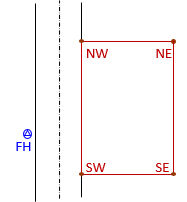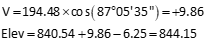### Problem 3

The diagram below represents a portion of a three-wire leveling circuit running from point BMR to point D to point M. Numbers on the diagram are the top, middle, and bottom wire readings for each sight. The elevation of BMR is 1245.36' and the instrument used has a stadia multiplier of 100.

Set up and complete the field notes for the circuit portion.#### Solution### Problem (1)

A TSI is set up 5.41' over point Q whose elevation is 745.39'. The following readings are taken:

 Point Horizontalangle Verticalangle Slopedistance 101 0°00'00" 4°24'10" 145.25' 102 39°56'15" -6°00'25" 89.32' 103 79°05'45" -2°58'30" 118.95'

The reflector height in each case was 5.64'. The instrument and reflector heights were measured with the same cloth tape.

What are the horizontal distances to and elevations of the observed points? Compute values to 0.01'.

#### Solution

Each vertical angle must be converted to a zenith angle.

##### Point 101Because the curvature and refraction is so small, it can be ignored for the other horizontal distances.Because the curvature and refraction is so small, it can be ignored for the other vertical distances.##### Point 102##### Point 103### Problem (2)

A TSI is set up over the SW corner of a property. The TSI height is 5.73' above the corner, measured with a fiberglass tape. The elevation of the top nut on a fire hydrant (FH) across the street is 827.43'.From the TSI, the following readings are taken

 Point ReflectorHeight1 Slopedistance Horizontalangle Zenithangle FH 5.00 84.39' 0°00'00" 95°30'45" NW 6.25 194.48' 44°51'55" 87°05'35" NE 6.25 240.68' 80°56'30" 85°48'05" SE 7.50 142.02' 134°51'35" 92°23'50"

1Measured with the same fiberglass tape.

What are the elevations of the four property corners? Compute to 0.01' to minimize rounding error.

#### Solution

We saw in the previous problem that over short distances, curvature and refraction are too small to affect elevations.

##### Point SW

Determine the elevation of the TSI at point SW from the fire hydrant, then the elevation of point SW from the TSI.##### Point NW##### Point NE##### Point SEEach solution is broken into discreet steps based on given elements. That makes it easier to see how the data relates and which computations must be performed.

### Problem (1)

The direction of line FG is S 47°24'30"W. The angle to the left at G from F to H is 71°50'05". What is the bearing of line GH?

 Direction of FG is S 47°24'30"W......angle to left at G from F to H is 71°50'05"......bearing of line GH:### Problem (2)

The azimuth of line KL is 125°00'20". The angle to the right at L from K from to M is 38°25'10". What is the azimuth of line LM?

 Azimuth of KL is 125°00'20"......angle right at L from K to M is 38°25'10"...(extending KL through L)...azimuth of LM:### Problem (3)

The azimuth of line CD is 205°05'30". The angle to the right at C from D to B is 122°18'10". What is the azimuth of line CB?

 Azimuth of CD is 205°05'30"...... angle right at C from D to B is 122°18'10"......azimuth of CB:### Problem (4)

The bearing of line ST is N 72°38'20"W. The deflection angle at S from T to U is 115°12'45"R. The angle to the left at U from T to V is 67°54'15". What is the bearing of line UV?

 Bearing ST is N 72°38'20"W.....defl angle at S from T to U is 115°12'45"R......angle left at U from T to V is 67°54'15"......bearing UV:### Problem (5)

The azimuth from A1 to B3 is 320°51'15". The bearing of line A1 to C4 is S 85°36'35"E. What is the angle to the right at A1 from B3 to C4?

 Azimuth A1 to B3 is 320°51'15"......bearing A1 to C4 is S 85°36'35"E......angle right at A1 from B3 to C4:### Problem (6)

The bearing from D5 to T6 is S 36°20'55"W. The bearing from T6 to L7 is N 58°25'40"W. What is the deflection angle at T6 from D5 to L7?

 Bearing D5 to T6 is S 36°20'55"W......bearing T6 to L7 is N 58°25'40"W......defl angle at T6 from D5 to L7:The answers (with the sig fig in each number underlined):

 Number Sig Fig Number Sig Fig Number Sig Fig 4.90 3 0.00120 3 0.001060 4 56.0020 6 000200.000 6 780. 3 0.0018 2 .0018 2 1.8x10-3 2

Note that the last row shows three different ways to express the same number to the same sig fig.

#### Part (a)

Length and bearing of the straight sides and arc chord traveling clockwise travel direction around the parcel.

Compute coordinates of all the points using intersections. In the process, lengths and directions of some sides will be determined. Inverse between coordinates for those that aren't.

(1) Compute coordinates of point Q(2) Using QB as a baseline, compute coordinates of TSolve angle at T using Law of Sines:Because angle T is greater than 90°,Angle at Q:Solve for LBT using the Law of SinesBearing BT:Coordinates of T from B:(3) Using QB as a baseline, compute coordinates of WSolve angle at B using Law of Cosines:Bearing BW:Coordinates of W from B:Math check - compute coordinates of W from Q (left to the reader).

(4) Using BW as a baseline, compute coordinates of KCompute the angle at B:Compute angle K from Law of Sines:Since K is less than 90°, it does not have to be subtracted from 180°.

Compute angle at W from angle conditionDetermine bearing W to K:Compute coordinates of K from W(5) Using coordinates, compute length and direction of Q to K(6) Using coordinates, compute chord T to Q length and directionLine Bearing Distance BT N21°50'31"W 155.42 TQ N49°23'51"E 170.42 QK S72°16'28"E 179.27 KW S4°50'13"W 214.57 WB N86°37'34"W 224.63

#### Part (b)

Central angle of curved side.

Line TQ is the arc chord - re-arrange the chord equation to solve the central angle#### Part c.

Parcel area to nearest 10 sq ft

Using coordinate method, compute area bounded by straight lines. To that, add the segment area a for curve TQ.

(1) Area by coordinates

 Point North East ( / ) ( \ ) T 244.26 442.18 157,049 Q 355.17 571.57 171,808 139,612 K 300.59 742.33 64,419 263,653 W 86.78 724.24 72,424.0 217,699 B 100.00 500.00 122,130. 43,390. T 244.26 442.18 44,218.0 587,830. 708,572(2) Segment areaTotal area = A1 + Aseg = 60,371+3074.5=63,445.5 = 63,450 sq ft